# How to check if every segments of a road have the same directions?

1077
17
11-02-2017 03:35 AM
Highlighted

Hi,

I’m attempting to make a linear referencing. It’s a polyline M layer (with lengths along every line)  of forest roads . Every road (unique road number) consists of 1, 2 or more segments. In the attribute table I have a field with segment_id (unique in the layer) and a field with the road_number (many segments can have the same road_number). The layer is sorted ascending by segment_id and road_number.

The problem is – how to check if every segments of one road_number have the same direction. Maybe the solution is to calculate distance for end point and start point of subsequent segments in every road_number (but not for first and last segments – only the middle segments). When the distance if more than 0 – it will be an error. But how to calculate it?

Thanks for any suggestions how to solve this.

Tags (1)
1 Solution

Accepted Solutions
Highlighted
byEsri Esteemed Contributor

You could try this. It will take the input fields to the output featureclass and add a field "FlipCase" that will hold "Flipped" when the polyline was flipped. However, the M values are not reversed for each point. Are the M values length values?

``def main():    import arcpy    import os    # input fc and name of field containing route name    fc = r'C:\GeoNet\RoadDirection2\test.gdb\roads_m'    fc_out = r'C:\GeoNet\RoadDirection2\test.gdb\roads_m_corr7' # output fc    fld_name = 'RouteName'    fld_id = 'Segment_ID'    fld_case = 'FlipCase'  # stores info on correction feature    tolerance = 10 # tolerance for searching connecting segments for same route    # sr    sr = arcpy.Describe(fc).spatialReference    arcpy.env.OverwriteOutput = True    # create dictionary of data    dct_routes = {}    dct_seg_id_oid = {}    dct_oid_seg_id = {}    flds = ('OID@', 'SHAPE@', fld_name, fld_id)    with arcpy.da.SearchCursor(fc, flds) as curs:        for row in curs:            dct_data = {}            oid = row            polyline = row            route_name = row            seg_id = row            dct_seg_id_oid[seg_id] = oid            dct_oid_seg_id[oid] = seg_id            if route_name in dct_routes:                dct_data = dct_routes[route_name]                dct_data[oid] = [polyline, seg_id]            else:                dct_data[oid] = [polyline, seg_id]            dct_routes[route_name] = dct_data    # supporting dct with oid and geometry    dct_oid_geom = {r: r for r in arcpy.da.SearchCursor(fc, ('OID@', 'SHAPE@'))}    # determnine first segment of route based on segment id    dct_start_seg_id = {}    for route_name, dct_data in sorted(dct_routes.items()):        seg_ids = [s for p,s in dct_data.items()]        dct_start_seg_id[route_name] = min(seg_ids)    # trace route from staring seg_id to end    lst_flip_oid = []    lst_candidates_todo = []    lst_multi_candidates = []    dct_route_order = {}    for route_name, seg_id in sorted(dct_start_seg_id.items()):        route_order = [seg_id]        print "trace:", route_name, " starting @", seg_id        dct_data = dct_routes[route_name]        dct_start_pnts = CreateDictionaryStartOrEndPoints(dct_data, "start")        dct_end_pnts = CreateDictionaryStartOrEndPoints(dct_data, "end")        oid = dct_seg_id_oid[seg_id]        start_pnt = dct_start_pnts[oid]        end_pnt = dct_end_pnts[oid]        lst_processed = [oid]        lst_todo = [o for o, lst in dct_data.items() if o != oid]        while len(lst_todo) > 0:            print "lst_processed:", lst_processed            # get candidates for end point of line            oid_cand_end, start_end_end, dist_from_end = GetCandidate(end_pnt, dct_start_pnts, dct_end_pnts, lst_todo, tolerance)            print " - GetCandidate 1:", oid_cand_end, start_end_end, dist_from_end            # get candidates for start point of line            oid_cand_start, start_end_start, dist_from_start = GetCandidate(start_pnt, dct_start_pnts, dct_end_pnts, lst_todo, tolerance)            print " - GetCandidate 2:", oid_cand_start, start_end_start, dist_from_start            # check is there are candidates            if dist_from_start == 9999 and dist_from_end == 9999:                # no candidates                print" - no candidates!"                lst_candidates_todo.extend(lst_todo)                lst_todo = []                oid_found = None            elif dist_from_start == dist_from_end:                # two candidates with same score, error!                print " - two candidates with same score, error!"                lst_multi_candidates.append([oid_cand_end, oid_cand_start])                lst_candidates_todo.extend(lst_todo)                lst_todo = []                oid_found = None            elif dist_from_start < dist_from_end:                # candidate is at start of line, requires flip of current oid                print " - candidate is at start of line, requires flip of current oid"                if start_end_start == "end":                    # also candidate need to be flipped                    print " - current start connects to candidate end, also candidate need to be flipped"                    print " - adding candidate oid ", oid_cand_start, " to flip list"                    lst_flip_oid.append(oid_cand_start)                if not oid in lst_flip_oid:                    lst_flip_oid.append(oid)                    print " - adding current oid ", oid, " to flip list"                oid_found = oid_cand_start            else:                # candidate is at end of line, OK                if start_end_end== "end":                    # current end connects to candidate end, candidate need to be flipped                    print " - current end connects to candidate end, candidate need to be flipped"                    lst_flip_oid.append(oid_cand_end)                    print "   - adding candidate oid ", oid_cand_end, " to flip list"                oid_found = oid_cand_end            if oid_found != None:                start_pnt = dct_start_pnts[oid_found]                end_pnt = dct_end_pnts[oid_found]                lst_processed.append(oid_found)                route_order.append(dct_oid_seg_id[oid_found])                lst_todo.remove(oid_found)                oid = oid_found            print "lst_todo", lst_todo            print "lst_flip_oid", lst_flip_oid        dct_route_order[route_name] = route_order        print    print "lst_flip_oid        :", sorted(lst_flip_oid)    print "lst_candidates_todo :", sorted(lst_candidates_todo)    print "lst_multi_candidates:", lst_multi_candidates    for route_name, route_order in sorted(dct_route_order.items()):        print " - Route:", route_name, "  seg id order:", route_order    # create output featureclass    ws, fc_name = os.path.split(fc_out)    arcpy.CreateFeatureclass_management(ws, fc_name, "POLYLINE", fc, "ENABLED", "DISABLED", sr)    arcpy.AddField_management(fc_out, fld_case, "TEXT", None, None, 50)    flds_in = [fld.name for fld in arcpy.ListFields(fc)]    flds_out = [fld.name for fld in arcpy.ListFields(fc_out)]    flds_in.remove('Shape_Length')    flds_out.remove('Shape_Length')    fld_geom = arcpy.Describe(fc).ShapeFieldName    fld_oid = arcpy.Describe(fc).OIDFieldName    flds_in[flds_in.index(fld_geom)] = 'SHAPE@'    i_oid = flds_in.index(fld_oid)    flds_in[flds_in.index(fld_oid)] = 'OID@'    flds_out[flds_out.index(fld_geom)] = 'SHAPE@'    flds_out[flds_out.index(fld_oid)] = 'OID@'    fld_geom = 'SHAPE@'    fld_oid = 'OID@'    cnt = 0    with arcpy.da.InsertCursor(fc_out, flds_out) as curs_out:        with arcpy.da.SearchCursor(fc, flds_in) as curs:            for row in curs:                cnt += 1                if cnt % 100 == 0:                    print "Processing feature:", cnt                oid = row[i_oid]                polyline = dct_oid_geom[oid] # row[i_shp]                if oid in lst_flip_oid:                    case = "Flipped"                    polyline_out = FlipPolyline(polyline)                else:                    case = "OK"                    polyline_out = polyline                row_out = []                for fld_name in flds_out[:-1]:                    row_out.append(row[flds_in.index(fld_name)])                row_out.append(case)                row_out[flds_out.index(fld_geom)] = polyline_out                print row_out                tpl_row = tuple(row_out)                curs_out.insertRow(tpl_row)def FlipPolyline(polyline):    print "before:", polyline.length    vertices = []    for part in polyline:        for pnt in part:            vertices.append(pnt)    polyline_out = arcpy.Polyline(arcpy.Array(vertices[::-1]),                polyline.spatialReference, False, True)    print "after :", polyline_out.length    return polyline_outdef GetCandidate(end_pnt, dct_start_pnts, dct_end_pnts, lst_todo, tolerance):    # return oid_cand_start, start_end, dist_from_start    dist_min = 9999    oid_min = None    type_min = None    for oid, pnt in dct_start_pnts.items():        if oid in lst_todo:            dist = GetDistance(end_pnt.X, end_pnt.Y, pnt.X, pnt.Y)            if dist < tolerance:                if dist < dist_min:                    dist_min = dist                    oid_min = oid                    type_min = "start"    for oid, pnt in dct_end_pnts.items():        if oid in lst_todo:            dist = GetDistance(end_pnt.X, end_pnt.Y, pnt.X, pnt.Y)            if dist < tolerance:                if dist < dist_min:                    dist_min = dist                    oid_min = oid                    type_min = "end"    return oid_min, type_min, dist_mindef GetDistance(x1, y1, x2, y2):    import math    return math.hypot(x2-x1, y2-y1)def CreateDictionaryStartOrEndPoints(dct_data, start_or_end):    dct_pnts = {}    for oid, lst in dct_data.items():        polyline = lst        if start_or_end == "start":            dct_pnts[oid] = polyline.firstPoint        else:            dct_pnts[oid] = polyline.lastPoint    return dct_pntsif __name__ == '__main__':    main()‍‍‍‍‍‍‍‍‍‍‍‍‍‍‍‍‍‍‍‍‍‍‍‍‍‍‍‍‍‍‍‍‍‍‍‍‍‍‍‍‍‍‍‍‍‍‍‍‍‍‍‍‍‍‍‍‍‍‍‍‍‍‍‍‍‍‍‍‍‍‍‍‍‍‍‍‍‍‍‍‍‍‍‍‍‍‍‍‍‍‍‍‍‍‍‍‍‍‍‍‍‍‍‍‍‍‍‍‍‍‍‍‍‍‍‍‍‍‍‍‍‍‍‍‍‍‍‍‍‍‍‍‍‍‍‍‍‍‍‍‍‍‍‍‍‍‍‍‍‍‍‍‍‍‍‍‍‍‍‍‍‍‍‍‍‍‍‍‍‍‍‍‍‍‍‍‍‍‍‍‍‍‍‍‍‍‍‍‍‍‍‍‍‍‍‍‍‍‍‍‍‍‍‍‍‍‍‍‍‍‍‍‍‍‍‍‍‍‍‍‍‍‍‍‍‍‍‍‍‍‍‍‍``
17 Replies
Highlighted
byEsri Esteemed Contributor

The are many ways to validate if there are segments with the wrong direction. For instance manually, by applying a symbology that includes line decoration: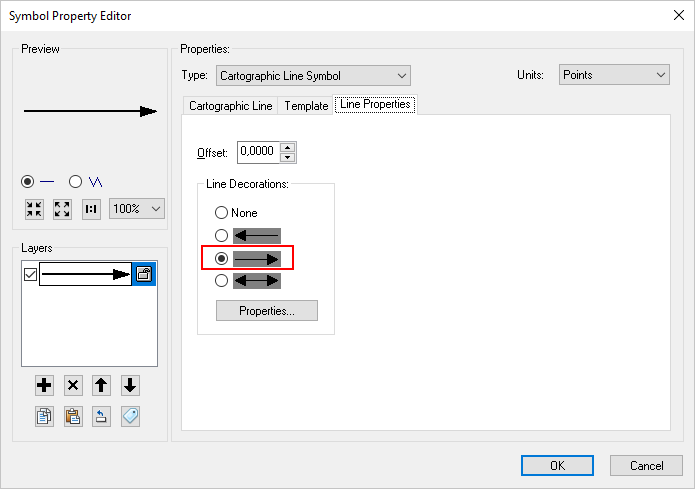Which will show you where you have problems in your data: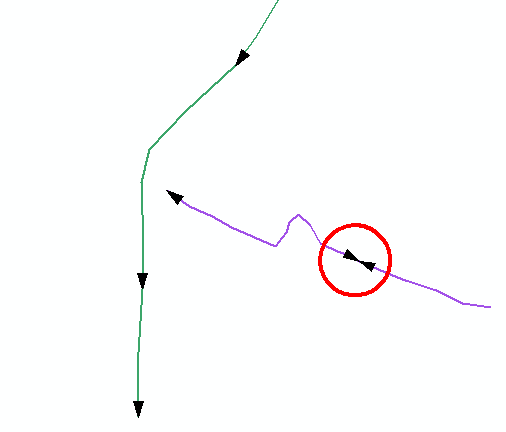You can also create end and start points in separate featureclasses form the lines using Feature Vertices to Points: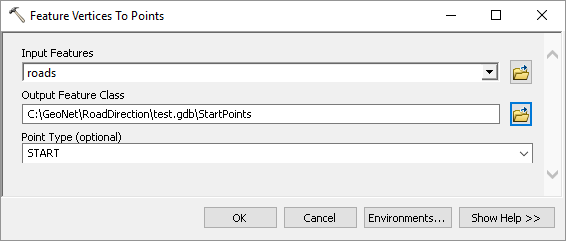And symbolize those: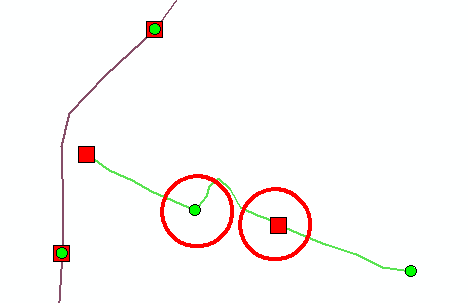You can also apply hatching (About displaying hatches—Help | ArcGIS Desktop ) to validate the M values of the road (are they continuous):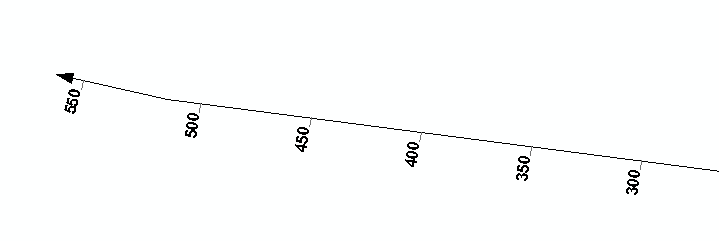Or you can analyze the start and end featureclasses with a Generate Near Table and validate where you have start points without end point (although you would need to exclude the start and end points of a route.

Or create routes to see if the routes makes sense when you apply the M values of the road segments.

Highlighted
Honored Contributor

Symbology > Arrow at End.Highlighted

Thank you for your answers and screenshots. Of course these solutions are good and I can apply them to my dataset.

But how to calculate (maybe in Python) distance for end point and start point of subsequent segments in every road_number (but not for first and last segments – only the middle segments) ? When the distance is more than 0 – it will be an error.

Highlighted
byEsri Esteemed Contributor

You can script this, but this can get pretty complex. I did something in the past. Let me dig into my archive and see if I can share some code.

Highlighted
byEsri Esteemed Contributor

See below some sample code that could be a start:

``def main():    import arcpy    # input fc and name of field containing route name    fc = r'C:\GeoNet\RoadDirection\test.gdb\roads_m'    fld_name = 'RouteName'    tolerance = 1    # sr    sr = arcpy.Describe(fc).spatialReference    # create dictionary of data    dct_routes = {}    flds = ('OID@', 'SHAPE@', fld_name)    with arcpy.da.SearchCursor(fc, flds) as curs:        for row in curs:            dct_data = {}            oid = row            polyline = row            route_name = row            if route_name in dct_routes:                dct_data = dct_routes[route_name]                dct_data[oid] = polyline            else:                dct_data[oid] = polyline            dct_routes[route_name] = dct_data    # determnine first segment of route based on minimum M value    dct_possible_errors = {}    for route_name, dct_data in sorted(dct_routes.items()):        print "dct_possible_errors", dct_possible_errors        oid = GetFirstSegmentOfRouteOnMvalue(dct_data)        print        print " - GetFirstSegmentOfRouteOnMvalue:", route_name, oid        lst_processed = [oid]        dct_start_pnts = CreateDictionaryStartOrEndPoints(dct_data, "start")        dct_end_pnts = CreateDictionaryStartOrEndPoints(dct_data, "end")        print route_name        print " - len:", len(dct_data.keys())        print " - oid:", oid        # start connecting segments        lst_todo = [o for o in dct_data.keys() if not o in lst_processed]        while len(lst_todo) != 0:            start_pnt = dct_start_pnts[oid]            end_pnt = dct_end_pnts[oid]            print " - lst_processed:", lst_processed            print " - lst_todo:", lst_todo            oid_near_end, dist_near_end, type_near_end = FindPointsNearEnd(oid, "end", dct_start_pnts, dct_end_pnts, lst_processed)            print " - FindPointsNearStartorEnd-end  :", oid_near_end, dist_near_end, type_near_end            oid_near_start, dist_near_start, type_near_start = FindPointsNearStart(oid, "start", dct_start_pnts, dct_end_pnts, lst_processed)            print " - FindPointsNearStartorEnd-start:", oid_near_start, dist_near_start, type_near_start            from_part = "end"            to_part = "not set"            if dist_near_start < tolerance:                print "   - connect to start is option..."                print "   - oid to connect to:", oid_near_start                to_part = "start"                print "ERROR possible flip line case"            if dist_near_end < tolerance:                print "   - connect to end is option..."                print "   - oid to connect to:", oid_near_end                to_part = "end"            print "    - from - to: ", from_part, " - ", to_part            if to_part == "end":                print "from_part is start, possible ERROR flip case!"                lst_todo.remove(oid_near_end)                lst_processed.append(oid_near_end)                oid = oid_near_end                print " - ERROR is end, Flip!:", oid_near_end                if route_name in dct_possible_errors:                    errors = dct_possible_errors[route_name]                    errors.append(oid)                    dct_possible_errors[route_name] = errors                else:                    dct_possible_errors[route_name] = [oid]                print " - added:", route_name, oid            elif to_part == "start":                print "to_part is end, OK"                lst_todo.remove(oid_near_start)                lst_processed.append(oid_near_start)                oid = oid_near_start                print " - OK is start        :", oid_near_start            else:                print "no candidates available in tolerance"                print " - still to do:", lst_todo                lst_todo = []    print    for route_name, errors in dct_possible_errors.items():        if len(errors) > 0:            print route_name, errorsdef FindPointsNearStart(oid, start_or_end, dct_start_pnts, dct_end_pnts, lst_exclude):    if start_or_end == "start":        pnt = dct_start_pnts[oid]    else:        pnt = dct_end_pnts[oid]    dist_min = 9999    oid_min = -1    type_min = "not set"    # determine distance bewteen point and start of others    for oid_i, pnt_i in dct_start_pnts.items():        if not oid_i in lst_exclude:            dist_i = GetDistance(pnt.X, pnt.Y, pnt_i.X, pnt_i.Y)            if dist_i < dist_min:                dist_min = dist_i                oid_min = oid_i                type_min = "start"    return oid_min, dist_min, type_mindef FindPointsNearEnd(oid, start_or_end, dct_start_pnts, dct_end_pnts, lst_exclude):    if start_or_end == "start":        pnt = dct_start_pnts[oid]    else:        pnt = dct_end_pnts[oid]    dist_min = 9999    oid_min = -1    type_min = "not set"   # determine distance bewteen point and start of others    for oid_i, pnt_i in dct_end_pnts.items():        if not oid_i in lst_exclude:            dist_i = GetDistance(pnt.X, pnt.Y, pnt_i.X, pnt_i.Y)            if dist_i < dist_min:                dist_min = dist_i                oid_min = oid_i                type_min = "end"    return oid_min, dist_min, type_mindef FindPointsNearStartorEnd(oid, start_or_end, dct_start_pnts, dct_end_pnts, lst_exclude):    if start_or_end == "start":        pnt = dct_start_pnts[oid]    else:        pnt = dct_end_pnts[oid]    dist_min = 9999    oid_min = -1    type_min = "not set"    # determine distance bewteen point and start of others    for oid_i, pnt_i in dct_start_pnts.items():        if not oid_i in lst_exclude:            dist_i = GetDistance(pnt.X, pnt.Y, pnt_i.X, pnt_i.Y)            if dist_i < dist_min:                dist_min = dist_i                oid_min = oid_i                type_min = "start"    # determine distance bewteen point and start of others    for oid_i, pnt_i in dct_end_pnts.items():        if not oid_i in lst_exclude:            dist_i = GetDistance(pnt.X, pnt.Y, pnt_i.X, pnt_i.Y)            if dist_i < dist_min:                dist_min = dist_i                oid_min = oid_i                type_min = "end"    return oid_min, dist_min, type_mindef GetDistance(x1, y1, x2, y2):    import math    return math.hypot(x2-x1, y2-y1)def CreateDictionaryStartOrEndPoints(dct_data, start_or_end):    dct_pnts = {}    for oid, polyline in dct_data.items():        if start_or_end == "start":            dct_pnts[oid] = polyline.firstPoint        else:            dct_pnts[oid] = polyline.lastPoint    return dct_pntsdef GetFirstSegmentOfRouteOnMvalue(dct_data):    m_min = 99999    oid_min = -1    for oid, polyline in dct_data.items():        for part in polyline:            for pnt in part:                m = pnt.M                if m < m_min:                    m_min = m                    oid_min = oid    return oid_minif __name__ == '__main__':    main()‍‍‍‍‍‍‍‍‍‍‍‍‍‍‍‍‍‍‍‍‍‍‍‍‍‍‍‍‍‍‍‍‍‍‍‍‍‍‍‍‍‍‍‍‍‍‍‍‍‍‍‍‍‍‍‍‍‍‍‍‍‍‍‍‍‍‍‍‍‍‍‍‍‍‍‍‍‍‍‍‍‍‍‍‍‍‍‍‍‍‍‍‍‍‍‍‍‍‍‍‍‍‍‍‍‍‍‍‍‍‍‍‍‍‍‍‍‍‍‍‍‍‍‍‍‍‍‍‍‍‍‍‍‍‍‍‍‍‍‍‍‍‍‍‍‍‍‍‍‍‍‍‍‍‍‍‍‍‍‍‍‍‍‍‍‍‍‍‍‍‍‍‍‍‍‍‍‍‍‍‍‍‍‍‍‍‍‍‍‍‍‍‍‍‍‍‍‍``

It threw as result that feature with OID 5, route "B" was in the wrong direction (although a lot of testing is still required):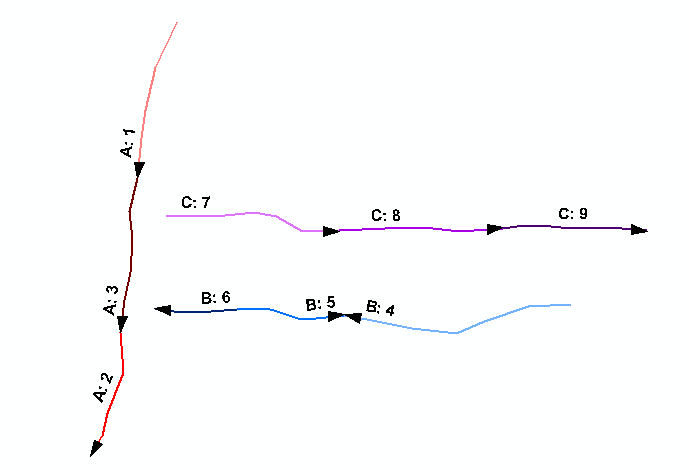Before you start to use this script, please keep the following in mind:

• This code selects the first segment (feature) based on the minimum M value
• The first feature defined the direction, if you have 10 segments in a route and the first points in a certain direction and the rest in the other, all 9 segments would be identified as "wrong"
• If you would like to define the correct direction based on the majority of the segments, you should determine if this is on number of features or combined length.
• There can be infinite exceptions that could yield undesired results
Highlighted

Thank you very much for your engagement and time you have spent on this case. I will apply and test the code. I will allow myself to contact you again in case of further questions.

Highlighted

I have done some test with your code on my example data. I have attached the data. You will find that:

• in my example data every segment of a given road must start with M value =0,
• the right direction is the direction of the first segment [Segment_ID] of a given road, that is the minimum value (number) in field [Segment_ID] for a given road number [RouteName]

Below screenshot of my example roads: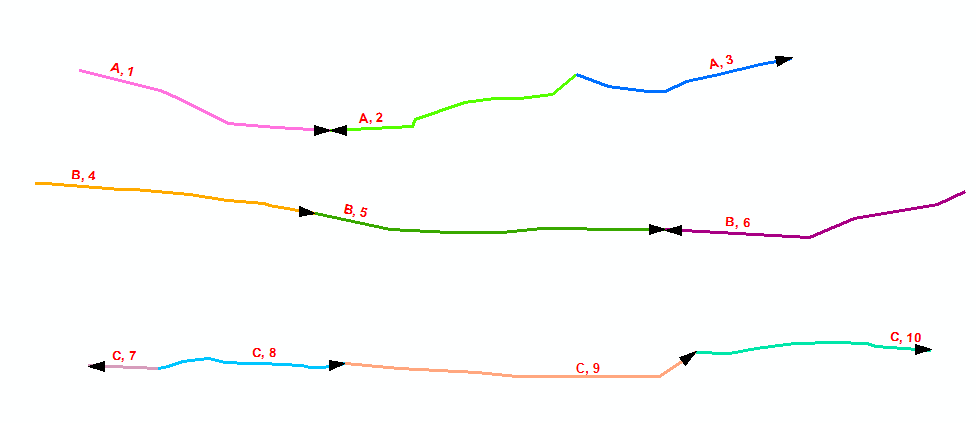The right direction for whole road indicates:

• Road A – segment 1
• Road B – segment 4
• Road C – segment 7

• Road A, Segment 2 (A, 2)
• Road B, Segment 6 (B, 6)
• Road C, Segment 8, 9, 10 (C,8; C,9; C,10)

For now, code indicates errors only at segment (A,2).

by